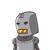# In swimming pool measuring 90 m X 40 m, 150 men take a dip. If the average displacement of water by a man is 8 m3 October 17, 2021 by Gianna

In swimming pool measuring 90 m X 40 m, 150 men take a dip. If the average displacement

of water by a man is 8 m3

, then rise in water level is​

### 1 thought on “In swimming pool measuring 90 m X 40 m, 150 men take a dip. If the average displacement <br /><br /> of water by a man is 8 m3<br”

1.Given;

Length of swimming pool= 90m=l

Displacement of water by 1 man = 8 cu.m.

To find;

Increase in height when 150 men take a dip in the pool (h)

Solution;

According to Archimedes principle,

Displacement of liquid = Total volume of body immersed in liquid.

Displacement of liquid = Increase in volume = l × b × h = 40*90*h

Volume of immersed body = Volume of 1 man * No. of men= 8*150

• ∴ 40 * 90 * h = 8 * 150
• 3600 * h = 1200
• h = 1200/3600 = 1/3
• h = 0.333 m.